# 1524292728760_Magnetic Properties of Compounds.pdf

May. 27, 2023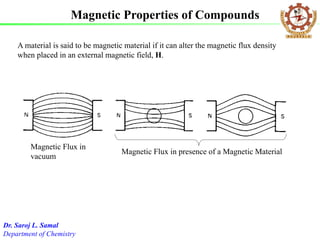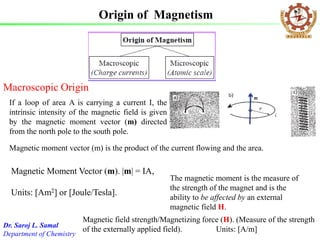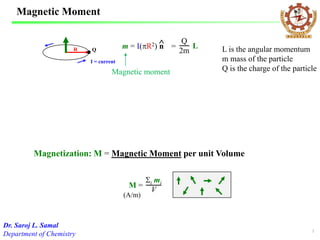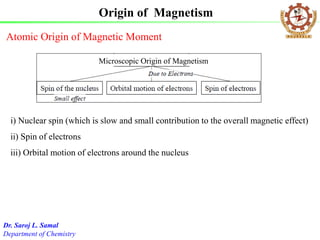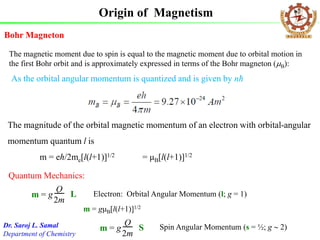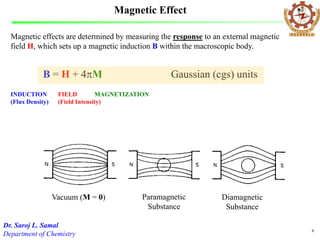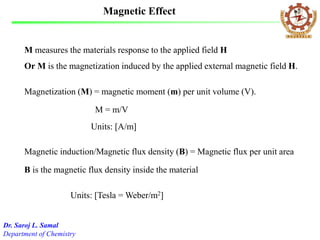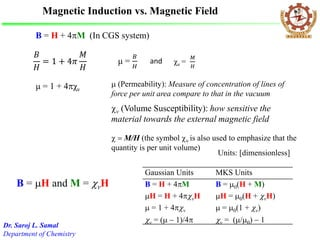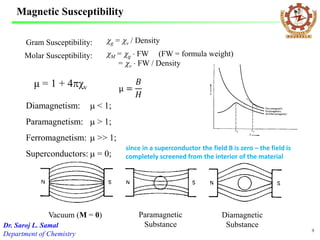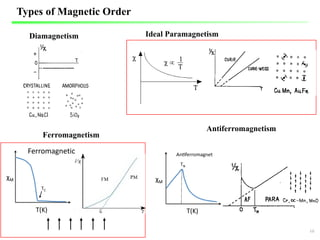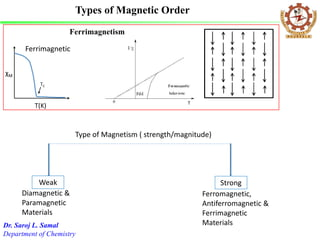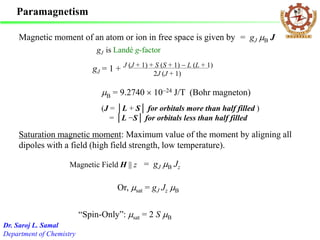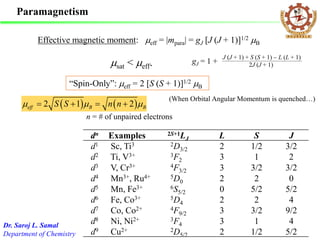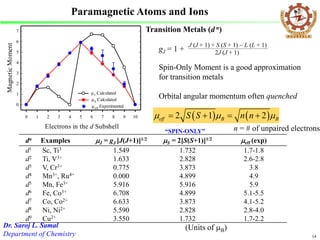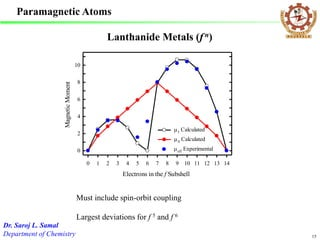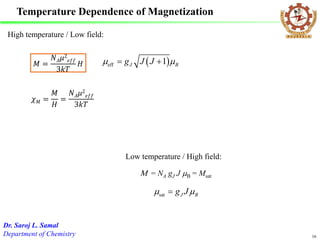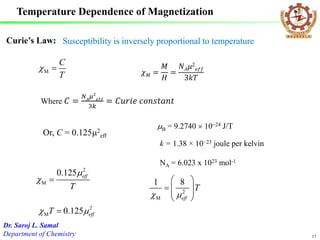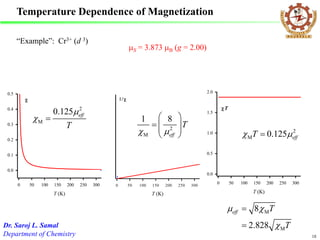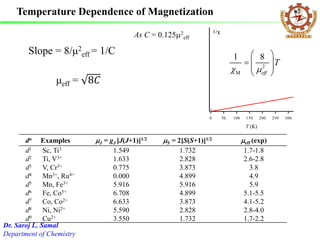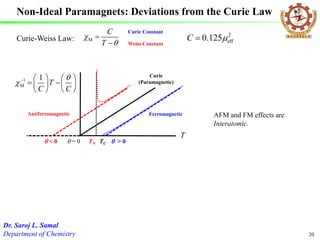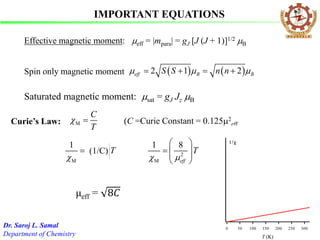1 of 21

### 1524292728760_Magnetic Properties of Compounds.pdf

• 1. A material is said to be magnetic material if it can alter the magnetic flux density when placed in an external magnetic field, H. Magnetic Flux in vacuum Magnetic Flux in presence of a Magnetic Material Magnetic Properties of Compounds Dr. Saroj L. Samal Department of Chemistry
• 2. Origin of Magnetism The magnetic moment is the measure of the strength of the magnet and is the ability to be affected by an external magnetic field H. Macroscopic Origin Magnetic Moment Vector (m). |m| = IA, Units: [Am2] or [Joule/Tesla]. Magnetic field strength/Magnetizing force (H). (Measure of the strength of the externally applied field). Units: [A/m] Dr. Saroj L. Samal Department of Chemistry If a loop of area A is carrying a current I, the intrinsic intensity of the magnetic field is given by the magnetic moment vector (m) directed from the north pole to the south pole. Magnetic moment vector (m) is the product of the current flowing and the area.
• 3. Magnetic Moment Magnetization: M = Magnetic Moment per unit Volume I = current R Q m = I(pR2) n  = L Q 2m M = Si mi V (A/m) 3 Dr. Saroj L. Samal Department of Chemistry L is the angular momentum m mass of the particle Q is the charge of the particle Magnetic moment
• 4. Atomic Origin of Magnetic Moment i) Nuclear spin (which is slow and small contribution to the overall magnetic effect) ii) Spin of electrons iii) Orbital motion of electrons around the nucleus Origin of Magnetism Dr. Saroj L. Samal Department of Chemistry Microscopic Origin of Magnetism
• 5. The magnitude of the orbital magnetic momentum of an electron with orbital-angular momentum quantum l is m = eℏ/2me[l(l+1)]1/2 = μB[l(l+1)]1/2 The magnetic moment due to spin is equal to the magnetic moment due to orbital motion in the first Bohr orbit and is approximately expressed in terms of the Bohr magneton (B): Origin of Magnetism Dr. Saroj L. Samal Department of Chemistry Bohr Magneton As the orbital angular momentum is quantized and is given by nħ m = g L Q 2m Electron: Orbital Angular Momentum (l; g = 1) Quantum Mechanics: m = gμB[l(l+1)]1/2 m = g S Q 2m Spin Angular Momentum (s = ½; g  2)
• 6. Magnetic Effect Magnetic effects are determined by measuring the response to an external magnetic field H, which sets up a magnetic induction B within the macroscopic body. B = H + 4pM Gaussian (cgs) units Vacuum (M = 0) Paramagnetic Substance Diamagnetic Substance INDUCTION (Flux Density) FIELD (Field Intensity) MAGNETIZATION 6 Dr. Saroj L. Samal Department of Chemistry
• 7. Magnetization (M) = magnetic moment (m) per unit volume (V). Magnetic induction/Magnetic flux density (B) = Magnetic flux per unit area Units: [Tesla = Weber/m2] B is the magnetic flux density inside the material M measures the materials response to the applied field H Or M is the magnetization induced by the applied external magnetic field H. M = m/V Magnetic Effect Dr. Saroj L. Samal Department of Chemistry Units: [A/m]
• 8. cv (Volume Susceptibility): how sensitive the material towards the external magnetic field B = H and M = cvH B = H + 4pM (In CGS system) 𝐵 𝐻 = 1 + 4𝜋 𝑀 𝐻 μ = 1 + 4pχv Gaussian Units MKS Units B = H + 4pM B = 0(H + M) H = H + 4pcvH H = 0(H + cvH)  = 1 + 4pcv  = 0(1 + cv) cv = ( - 1)/4p cv = (/0) - 1 Magnetic Induction vs. Magnetic Field c  M/H (the symbol cv is also used to emphasize that the quantity is per unit volume) Dr. Saroj L. Samal Department of Chemistry  (Permeability): Measure of concentration of lines of force per unit area compare to that in the vacuum Units: [dimensionless] μ = 𝐵 𝐻 χv = 𝑀 𝐻 and
• 9. Magnetic Susceptibility Gram Susceptibility: cg = cv / Density Molar Susceptibility: cM = cg  FW (FW = formula weight) = cv  FW / Density 9 μ = 1 + 4pχv μ = 𝐵 𝐻 Dr. Saroj L. Samal Department of Chemistry Vacuum (M = 0) Paramagnetic Substance Diamagnetic Substance Diamagnetism:  < 1; Paramagnetism:  > 1; Ferromagnetism:  >> 1; Superconductors:  = 0; since in a superconductor the field B is zero – the field is completely screened from the interior of the material
• 10. Diamagnetism Ideal Paramagnetism Ferromagnetism Antiferromagnetism Types of Magnetic Order 10 Ferromagnetic
• 11. Ferrimagnetism Type of Magnetism ( strength/magnitude) Weak Strong Diamagnetic & Paramagnetic Materials Ferromagnetic, Antiferromagnetic & Ferrimagnetic Materials Ferrimagnetic Types of Magnetic Order Dr. Saroj L. Samal Department of Chemistry
• 12. Magnetic moment of an atom or ion in free space is given by = gJ B J B = 9.2740  10-24 J/T (Bohr magneton) (J = │L + S│ for orbitals more than half filled ) = │L −S│ for orbitals less than half filled gJ = 1 + J (J + 1) + S (S + 1) - L (L + 1) 2J (J + 1) Paramagnetism gJ is Landé g-factor Saturation magnetic moment: Maximum value of the moment by aligning all dipoles with a field (high field strength, low temperature). Or, sat = gJ Jz B “Spin-Only”: sat = 2 S B Magnetic Field H || z = gJ B Jz Dr. Saroj L. Samal Department of Chemistry
• 13. Paramagnetism Effective magnetic moment: eff = |mpara| = gJ [J (J + 1)]1/2 B “Spin-Only”: eff = 2 [S (S + 1)]1/2 B (When Orbital Angular Momentum is quenched…) sat < eff. Dr. Saroj L. Samal Department of Chemistry gJ = 1 + J (J + 1) + S (S + 1) - L (L + 1) 2J (J + 1) dn Examples 2S+1LJ L S J d1 Sc, Ti3 2D3/2 2 1/2 3/2 d2 Ti, V3+ 3F2 3 1 2 d3 V, Cr3+ 4F3/2 3 3/2 3/2 d4 Mn3+, Ru4+ 5D0 2 2 0 d5 Mn, Fe3+ 6S5/2 0 5/2 5/2 d6 Fe, Co3+ 5D4 2 2 4 d7 Co, Co2+ 4F9/2 3 3/2 9/2 d8 Ni, Ni2+ 3F4 3 1 4 d9 Cu2+ 2D5/2 2 1/2 5/2     2 1 2 eff B B S S n n        n = # of unpaired electrons
• 14. Electrons in the d Subshell 0 1 2 3 4 5 6 7 8 9 10 Magnetic Moment 0 1 2 3 4 5 6 7 J Calculated S Calculated eff Experimental Spin-Only Moment is a good approximation for transition metals Orbital angular momentum often quenched gJ = 1 + J (J + 1) + S (S + 1) - L (L + 1) 2J (J + 1) 14 Transition Metals (d n) Dr. Saroj L. Samal Department of Chemistry Paramagnetic Atoms and Ions dn Examples J = gJ [J(J+1)]1/2 S = 2[S(S+1)]1/2 eff (exp) d1 Sc, Ti3 1.549 1.732 1.7-1.8 d2 Ti, V3+ 1.633 2.828 2.6-2.8 d3 V, Cr3+ 0.775 3.873 3.8 d4 Mn3+, Ru4+ 0.000 4.899 4.9 d5 Mn, Fe3+ 5.916 5.916 5.9 d6 Fe, Co3+ 6.708 4.899 5.1-5.5 d7 Co, Co2+ 6.633 3.873 4.1-5.2 d8 Ni, Ni2+ 5.590 2.828 2.8-4.0 d9 Cu2+ 3.550 1.732 1.7-2.2 (Units of B) “SPIN-ONLY”     2 1 2 eff B B S S n n        n = # of unpaired electrons
• 15. Paramagnetic Atoms Electrons in the f Subshell 0 1 2 3 4 5 6 7 8 9 10 11 12 13 14 Magnetic Moment 0 2 4 6 8 10 J Calculated S Calculated eff Experimental Must include spin-orbit coupling Largest deviations for f 5 and f 6 15 Lanthanide Metals (f n) Dr. Saroj L. Samal Department of Chemistry
• 16. Temperature Dependence of Magnetization   eff 1 J B g J J     16 𝑀 = 𝑁𝐴𝜇2 𝑒𝑓𝑓 3𝑘𝑇 𝐻 𝜒𝑀 = 𝑀 𝐻 = 𝑁𝐴𝜇2 𝑒𝑓𝑓 3𝑘𝑇 Dr. Saroj L. Samal Department of Chemistry High temperature / Low field: Low temperature / High field: M = NA gJ J B = Msat sat J B g J   
• 17. Temperature Dependence of Magnetization 17 Curie’s Law: M C T c  Where 𝐶 = 𝑁𝐴 𝜇2 𝑒𝑓𝑓 3𝑘 = 𝐶𝑢𝑟𝑖𝑒 𝑐𝑜𝑛𝑠𝑡𝑎𝑛𝑡 Susceptibility is inversely proportional to temperature Or, C = 0.1252 eff 2 M 0.125 eff T  c  2 M 0.125 eff T c   2 M 1 8 eff T c           Dr. Saroj L. Samal Department of Chemistry 𝜒𝑀 = 𝑀 𝐻 = 𝑁𝐴𝜇2 𝑒𝑓𝑓 3𝑘𝑇 B = 9.2740  10-24 J/T k = 1.38 × 10−23 joule per kelvin NA = 6.023 x 1023 mol-1
• 18. Temperature Dependence of Magnetization T (K) 0 50 100 150 200 250 300 0.0 0.1 0.2 0.3 0.4 0.5 T (K) 0 50 100 150 200 250 300 T (K) 0 50 100 150 200 250 300 T 0.0 0.5 1.0 1.5 2.0 2 M 0.125 eff T  c  2 M 1 8 eff T c           2 M 0.125 eff T c   “Example”: Cr3+ (d 3) S = 3.873 B (g = 2.00) 18 M M 8 2.828 eff T T  c c   Dr. Saroj L. Samal Department of Chemistry
• 19. Slope = 8/μ2 eff = 1/C μeff = 8𝐶 T (K) 0 50 100 150 200 250 300 2 M 1 8 eff T c           As C = 0.1252 eff dn Examples J = gJ [J(J+1)]1/2 S = 2[S(S+1)]1/2 eff (exp) d1 Sc, Ti3 1.549 1.732 1.7-1.8 d2 Ti, V3+ 1.633 2.828 2.6-2.8 d3 V, Cr3+ 0.775 3.873 3.8 d4 Mn3+, Ru4+ 0.000 4.899 4.9 d5 Mn, Fe3+ 5.916 5.916 5.9 d6 Fe, Co3+ 6.708 4.899 5.1-5.5 d7 Co, Co2+ 6.633 3.873 4.1-5.2 d8 Ni, Ni2+ 5.590 2.828 2.8-4.0 d9 Cu2+ 3.550 1.732 1.7-2.2 Temperature Dependence of Magnetization Dr. Saroj L. Samal Department of Chemistry
• 20. Non-Ideal Paramagnets: Deviations from the Curie Law M C T c   - Curie-Weiss Law: Curie Constant Weiss Constant 1 M 1 T C C  c-      -          = 0  > 0  < 0 TN T TC Antiferromagnetic Ferromagnetic 2 eff 0.125 C   AFM and FM effects are Interatomic. 20 Curie (Paramagnetic) Dr. Saroj L. Samal Department of Chemistry
• 21. Effective magnetic moment: eff = |mpara| = gJ [J (J + 1)]1/2 B     2 1 2 eff B B S S n n        Spin only magnetic moment Curie’s Law: M C T c  (C =Curie Constant = 0.1252 eff 2 M 1 8 eff T c           μeff = 8𝐶 T (K) 0 50 100 150 200 250 300 2 M 1 8 eff T c           (1/C) IMPORTANT EQUATIONS Saturated magnetic moment: sat = gJ Jz B Dr. Saroj L. Samal Department of Chemistry